# Grinding Media Wear Rate Calculation in Ball Mill

In the previous discussion the fact was established that the work done by a ball when it strikes at the end of its parabolic path is proportional to its weight and velocity; then, since the velocity may be considered as constant for all the balls in the mill, the work done by a ball is proportional to its weight. Since the amount of ore crushed varies as the work done upon it, it seems reasonable that the amount of steel worn from the balls varies as the work done upon them; in other words, the ball wear is proportional to the work done. But it has been shown that the work done is proportional to the weight of the ball; hence, the wear is proportional to the weight of the ball, or

R = KW……………………………………..(23)

in which R is the rate of wear of any ball of weight W; K is a constant and depends upon the operating conditions of the mill and the resistance of the material from which the balls are made. In order to establish the accuracy of this equation, some transformations are necessary.

After a ball-mill has been in continuous and steady operation for, say, a year, during which time balls of only one diameter have been added at regular intervals, it may be assumed that the ball charge has reached a constant working condition. In this constant state, there is no change in the charge from day to day, either as to the weight of the charge or the average diameter of the balls composing it. Any particular ball enters the mill at a maximum diameter D, and gradually wears down until it is completely worn away; the residual charge of balls, however, always remains the same.

Suppose these balls composing the residual charge to be laid out in a line, beginning with the largest and ending with the smallest. Suppose intervals to be marked off along this line so that all of the balls of diameter from Dm to D1 are in the first interval, from D1 to D2 in the second interval, D2 to D3 in the third interval, and so on. Let N1, N2, N3, etc., represent the number of balls in each interval, and let D’1, D’2, D’3, etc., represent the mean diameters of the balls in their respective intervals.

In the constant state, the number of balls in each of these intervals does not vary, and when a new ball of diameter Dm is added to the first interval, a ball of diameter D1 passes into the second interval, and so on down the line, until in the last interval one ball is entirely worn away. Then, as time goes on, each ball of diameter Dm which was added gradually passes along the line until its diameter becomes D1 when it passes from the first interval into the second. If in time T, Nw balls of diameter Dm are added, then Nw balls will pass from each interval into the next following interval. The time T required for any ball to pass through any interval may then be expressed by the formula,

T = N’t/Nw

or the time required for any ball to reduce its diameter from Da to Db is equal to the total number of balls in the interval divided by the number of balls added to the intervals, or passing from the interval during this time.

If, as previously suggested, the wear varies as the weight of the ball, then R = KW = Kπ/6SD³, in which S is the weight of the material from which the balls are made and D is the diameter of the ball in question.

This formula shows the rate of wear at any instant. Since rate is always equal to the differential of space with respect to time, then R = dw/dt in which dw is the weight of material worn off in a very small interval of time dt.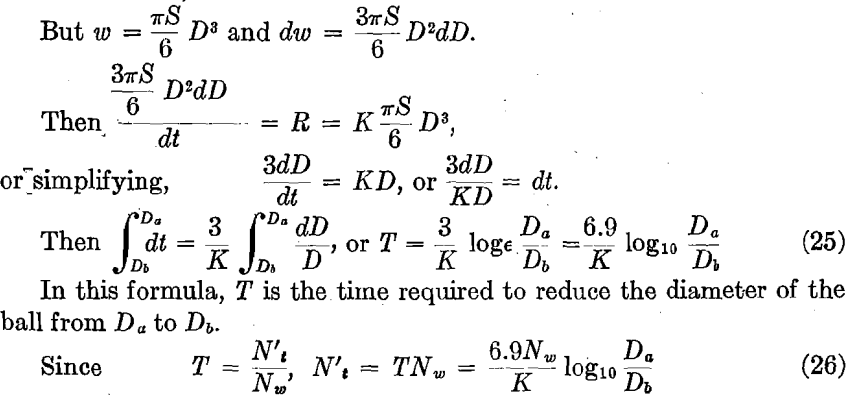This formula gives the total number of balls of diameter between Da and Db.

The rate of wear is equal to the amount worn off divided by the time required; but the amount worn off in any interval is 1/6πS(Da³ – Db³).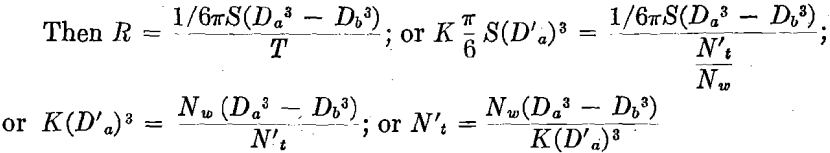The total weight of the balls in any interval is equal to the number of balls in the interval times the mean weight of these balls,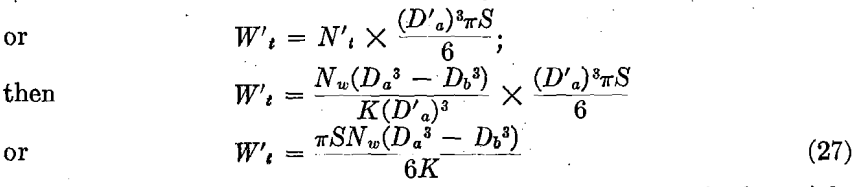But the sum of the weights of the balls in each section is the weight of the entire ball charge, or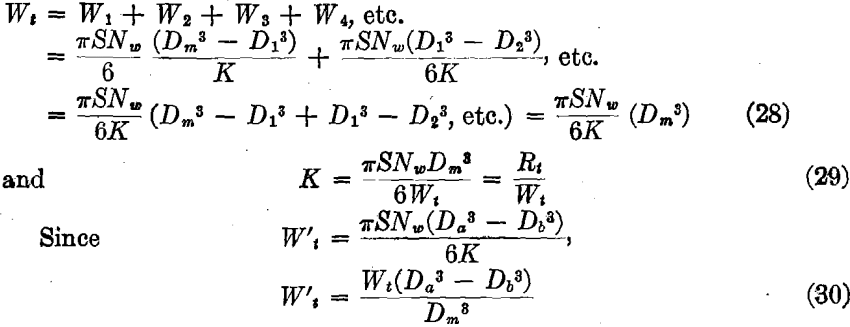which is the weight of balls in one interval. The per cent, weight of balls in an interval is given by the formula,

% W’t = Da³ – Db³/Dm³ x 100………………………………..(31)

The average weight of the balls of diameter between Da and Db is equal to the total weight of the balls divided by the total number of balls, or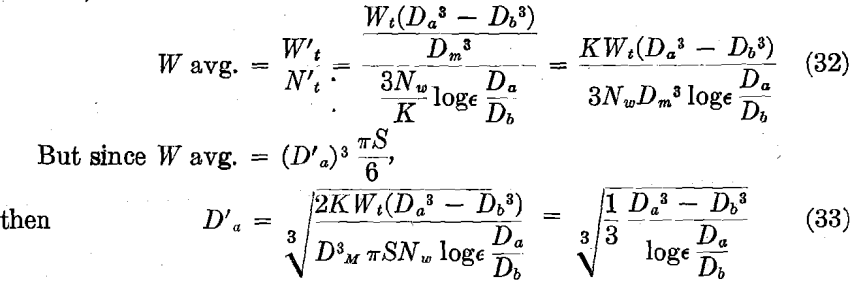By use of formula (31) it is possible to compute the actual screen analysis of the balls in the mill. If the balls are removed from the mill when they reach a certain minimum diameter, D0, formula (31) becomes,

% W’t = Da³ – Db³/Dm³ – Do³……………………………(34)

in which Da and Db are the upper and lower limits of the diameter for any desired interval, Dm is the diameter of the balls charged to the mill, and Do is the diameter below which the balls are removed from the mill.

It should be remembered, however, that these formulas (31) and (34) hold true only provided that the balls wear down at a rate proportional to their weight. It would seem that if the percentage weight computed by formulas (31) and (34) agreed reasonably closely with the actual results secured by carefully screening a ball charge after the mill had been in operation a sufficient length of time for the charge to become steady, and if this agreement could be secured in a number of cases under different conditions, it would be convincing evidence that the ball wear varies with the weight of the ball.

## Data on Ball Charges

In an endeavor to follow this plan, the attempt has been made to secure reliable data showing the screen analysis of ball charges which have been in steady use for a long period. It seems to be difficult to secure reliable information on this subject but the following results seem to indicate the truth of this law of ball wear.

The screen analysis of the ball charge of an 8-ft. by 22-in. (2.4-m. by 55.8-cm.) Hardinge conical mill shown in Table 19 was made at the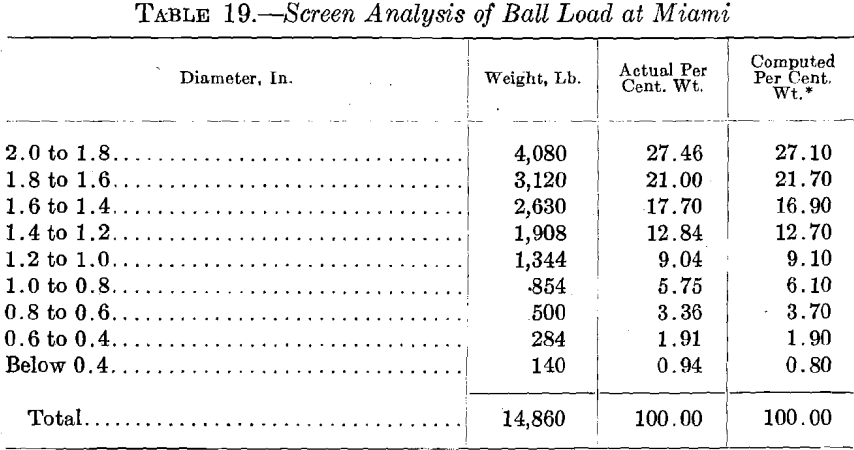Miami Copper Co.’s plant after the mill had been operating for a year with a ball load of 14,800 lb. (6713 kg.) which was maintained by the addition of 400 lb. (181 kg.) of 2-in. (50.8-mm.) steel balls daily.

In this table, the actual per cent, weight obtained by weighing the balls is compared with the theoretical per cent, weight computed by use of formula (30). The two columns of figures are almost identical, thereby showing the accuracy of the formula and the truth of the law of ball wear.

At the Golden Cycle Mining and Reduction Co.’s plant a dry crushing test was made on a 6 ft. 2 in. by 6-ft. (1.85 by 1.8-m.) Kominuter ball-mill. The mill was operated for 694 hr., during which time 4825 lb. (2188 kg.) of 5½-in. (139.7-mm.) balls were added. The original ball load in the mill was 6614 lb. (3000 kg.) and the load at the end of the 694 hr. was 6338 lb. (2874.8 kg.). During this time, 590 lb. (267.6 kg.) of balls less than 3 in. (76.2 mm.) in diameter were discarded from the mill. The screen analysis of the ball charge at the end of the operation is shown in Table 20.

The slight irregularity in these results may be explained by the fact that at one time during the test, after about 400 hr., twenty-two 5½-in. balls were added at one time. This may explain the irregularities at about 5-in. size.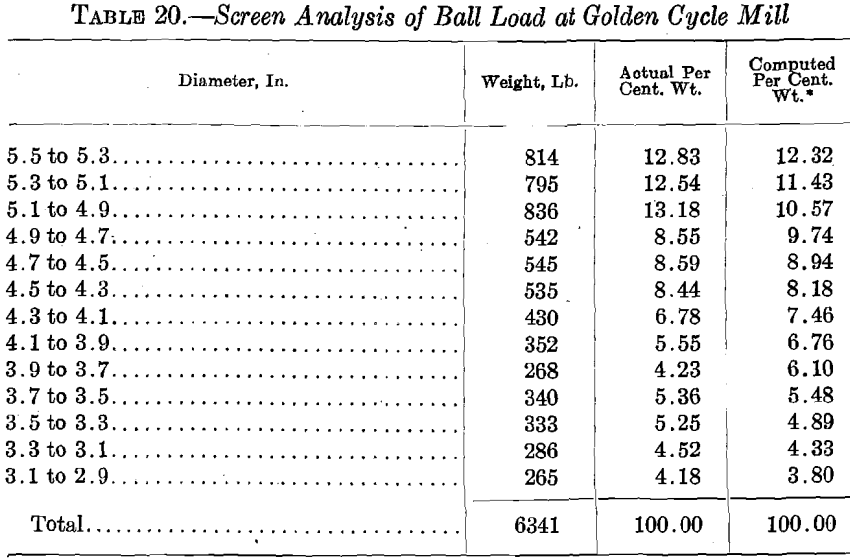From the two tests reported, the agreement between the actual per cent, weight and the computed per cent, weight is as close as could be expected. One was on a wet-crushing conical mill, and the other was on a dry-crushing cylindrical mill, and as far as the available data are concerned, the law of ball wear seems to be proved. It may develop however, when more data are collected, that the wear, instead of being proportional to the cube of the diameter, will be proportional to some slightly higher or lower power.

## Ball Mill Ball Wear Projection

1. In any mill, the rate at which the weight of any ball decrease is directly proportional to its weight.
2. In any mill, the rate at which the diameter of a ball decreases is directly proportional to its diameter.
3. In any mill, the rate at which the surface of any ball decreases is proportional to its surface.
4. Since the rate at which a ball loses weight varies as the work done upon it in the mill, it follows that the work done in wearing (or crushing) the ball varies as the weight of the ball. This is seen to be Kick’s law.
5. It then appears that Kick’s law holds true for the ball wear in a rotating mill.
6. The natural tendency is for the small balls to accumulate in the mill charge.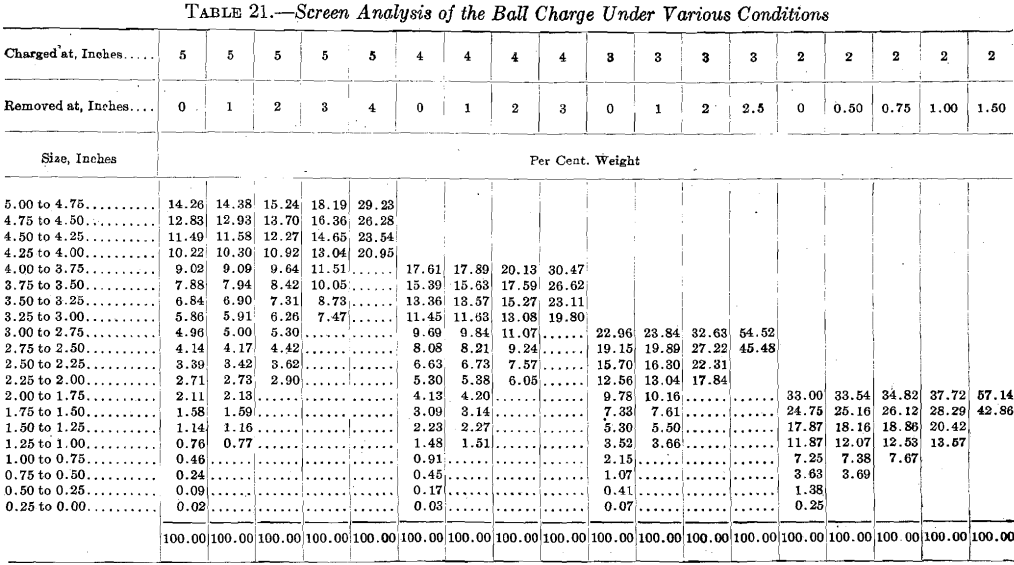7. Since these small balls do very little crushing, and exclude ore and larger balls from the mill, if allowed to accumulate too long, a marked decrease in crushing efficiency will result.
8. Since the large ball is just as likely to strike the small pieces of ore and the small ball is just as likely to strike the large pieces of ore as the reverse, it would seem that all of the balls should be of a size to crush any of the particles of ore.
9. This means that the balls should be as nearly as possible of a uniform size.
10. Since spheres of uniform size provide the greatest amount of interstitial space, a mill charge composed of balls of uniform size will allow freer migration of the ore particles than a charge containing balls of different sizes.

## Recapitulation of Ball Wear Formulas

Nw = number of balls added in time T to compensate for the ball wear.
Nt = total number of balls in the mill.
N’t = total number of balls in any interval.
N1, N2, N3, etc. = number of balls in the first, second, third, etc., intervals.
D = diameter of any ball under consideration.
Dm = diameter of balls added to compensate for wear.
D’a = mean diameter of balls in any interval.
Da = diameter at beginning of interval.
Db = diameter at end of interval.
D1, D2, D3, etc. = diameter of balls at end of first, second, third, etc., intervals.
Wt = total weight of balls in the mill,
W’t = total weight of balls in any interval.
w = weight of any ball.
R = rate of ball wear.
Rt = loss in weight of the mill charge in time T. It is equal to π/6Dm³ SNw.
T = time required for any ball to pass through any interval.
S = weight of material from which balls are made.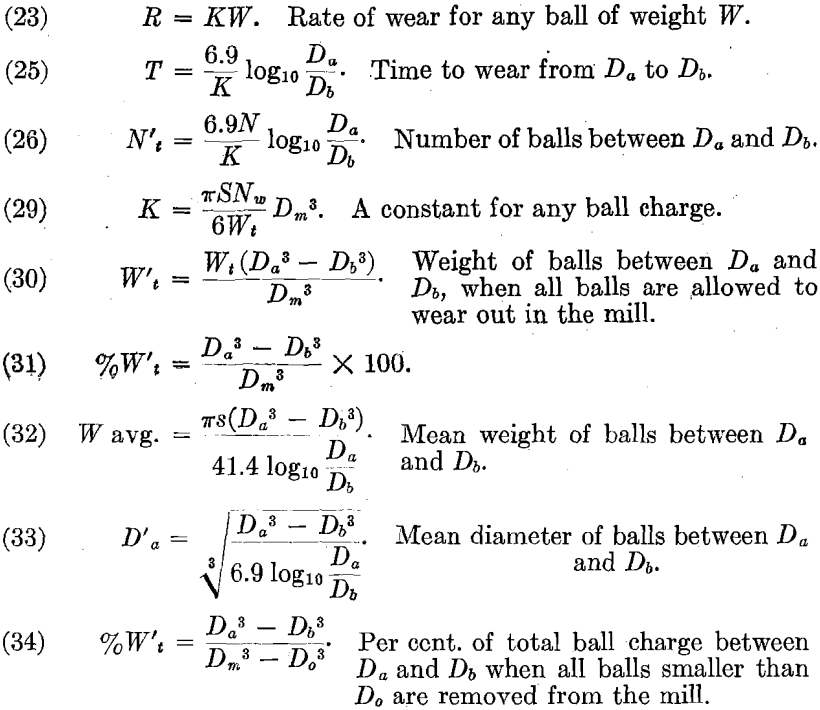## Practical Application of Theoretical Conclusions

The question finally arises as to how fine-crushing practice can be improved by the application of any of the principles that have been set forth. It is felt that the chief benefit to the mill operator will be derived from the fact that he may be better acquainted with exactly what is going on inside the ball-mill under various conditions. He should have a mental picture of the action of the charge and know better how to correct the difficulties encountered. He should also have a better idea how to proceed in order to produce any desired result.

While it is possible mathematically, as has been shown, to calculate the proper mill speed for any definite volume of charge, the size of the balls to be used must be determined experimentally. The size of balls is a most important factor in crushing, and each different condition requires balls of a different size. Whether the ore is hard or soft, coarse or fine, does not affect the proper mill speed or the volume of the charge; these depend almost entirely upon the size, and possibly to some small extent on the characteristics of the mill. But the proper size of the balls can be determined only by a careful study of the existing conditions. Experimental data must here be resorted to and the following method is recommended as a good means for determining the exact size of the balls that should be used.

Charge the mill with large balls, say of 5-in. (127 mm.) diameter. A smaller size might be better, but balls should be used that are known to be too large. An ammeter or wattmeter should be connected in the circuit of the driving motor so that the operator may keep the ball load constant by observing the power required by the mill. For maintaining this constant ball load, only balls of the same diameter as are already in the mill should be added. That is, if the test is started with all 5-in. balls, at the end of 24 hr. these balls will all be, say 4¾ in.; the ball load should then be restored to its original weight by adding only 4¾- in. balls. Thus, each time balls are added a different size must be used.

In this manner the mill will be filled with balls of approximately uniform diameter at all times. Then by keeping the records of each day’s run, it will be possible for the operator to determine just which size produced the best results under the conditions at hand. The ball charge should then be composed of balls as near this size as is practical and economical.

There are two methods of determining the proper size of the balls to be added at the end of each 24 hr. One method is to sample the ball charge and actually measure the balls. In some types of mills a sampler can be inserted through the discharge trunnion. If this is not possible the size of the balls can be computed, but samples should be obtained at certain intervals in order to check the computations. The method of computing the ball size comes directly from the ball-wear formula and is, as follows:

First determine the ball wear for the previous 24 hr. This may be done by obtaining a rough calibration of the power meter in the motor circuit so that any definite decrease in power indicates a given decrease in the weight of the charge. This is, then, the ball wear in pounds per day.

Then in ball-wear formula (25), T = 6.9/K Log10 Da/Db; but from (29), K = Rt/Wt. Then T = 6.9Wt/Rt Log10 Da/Db T is 1 day, Wt is the original weight of the ball charge, and Rt is the ball wear for one day. Then Log10 Da/Db = Rt/6.9Wt are all known, and it is only necessary to solve for Db, the diameter of the balls to be added. If only Rt lb. of these balls are added, then any error in computing Rt will not accumulate but will be corrected on the following day.

The above method for determining the proper size of the balls will, of course, require the careful attention of some one outside the ordinary mill crew. It also calls for a large assortment of balls of various diameters. As compensation for the trouble and expense necessary for the proper carrying out of this experiment, the operator stands a good chance of increasing the ball-mill capacity by a very considerable amount.

Once the proper size of balls is determined, the charge should be maintained so that it is composed of balls as little larger and as little smaller than the average diameter ball as is possible. Just how closely the proper ball charge can be maintained depends upon the facilities and economic conditions at the plant. The removal of the small balls, which is the main difficulty, is not so serious as it may seem at first; proper equipment makes this easy and inexpensive. It is to be hoped that the makers of ball-mills will succeed in producing a mill that will automatically discard balls of any desired diameter as rapidly as they are formed.

Another point which will bear investigation is the classifier capacity. As has been pointed out, large circulating loads seem essential for best efficiency. Classification is cheap compared with fine crushing and a classification capacity in excess of the capacity of the ball-mill is very much to be desired.

Acknowledgment is made for permission to publish data to the managers of this Mesabi Syndicate and allied interests under whose direction this work was done; to B. B. Gottsberger, General Manager of the Miami Copper Co., Miami, Ariz.; to A. L. Blomfield, General Manager of the Golden Cycle Mining and Reduction Co., Colorado Springs, Colo., who furnished valuable data; and to W. G. Swart, Fred A. Jordan and T. B. Counselman, at Duluth, who assisted in the experimental work and in the compilation of results.